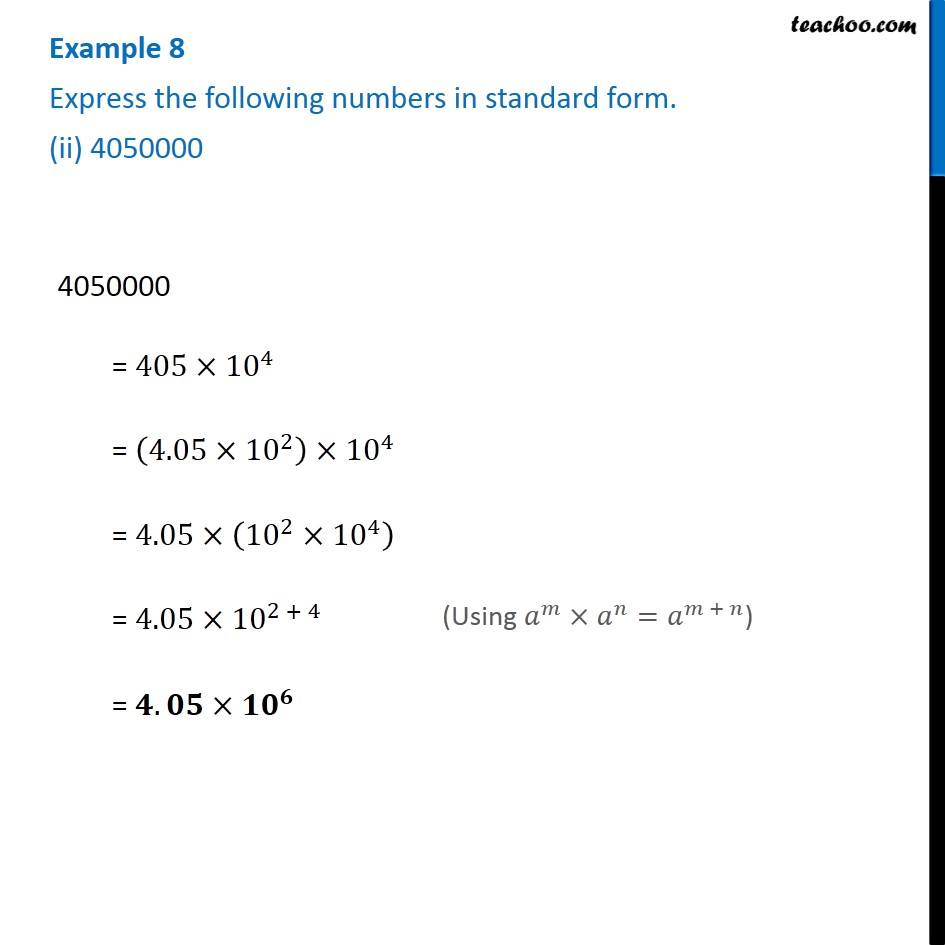1. Chapter 12 Class 8 Exponents and Powers
2. Serial order wise
3. Examples

Transcript

Example 8 Express the following numbers in standard form. (ii) 4050000 4050000 = 405×〖10〗^4 = (4.05×〖10〗^2 )×〖10〗^4 = 4.05×(〖10〗^2×〖10〗^4 ) = 4.05×〖10〗^(2 + 4) = 𝟒.𝟎𝟓×〖𝟏𝟎〗^𝟔 (Using 𝑎^𝑚×𝑎^𝑛=𝑎^(𝑚 + 𝑛))